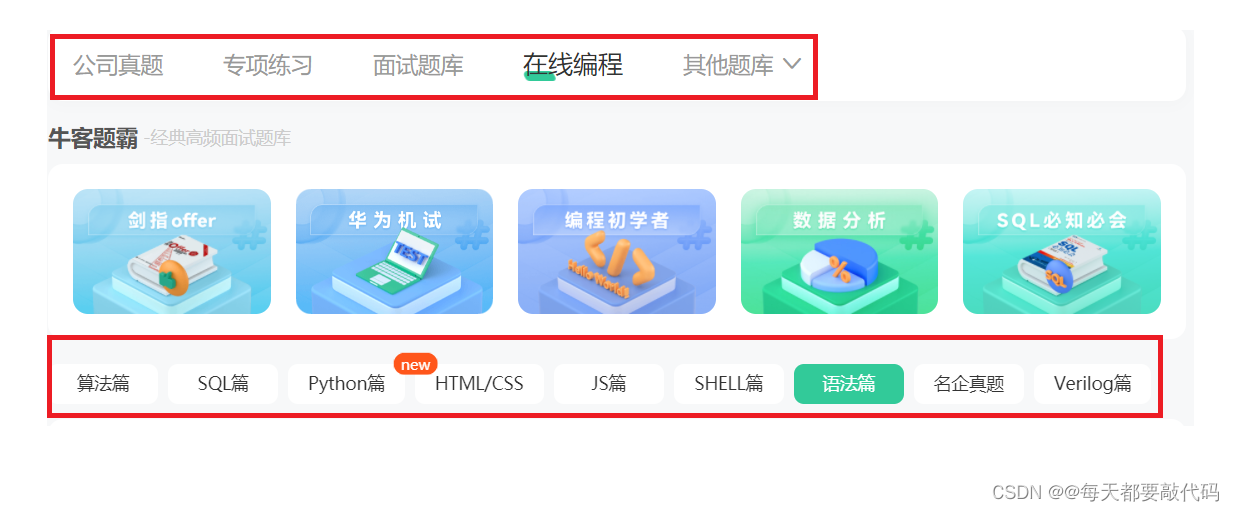> 文档中心 > 带你刷（牛客网）C语言百题（第十六天）

# 带你刷（牛客网）C语言百题（第十六天）

✅作者简介：大家好我是@每天都要敲代码，希望一起努力，一起进步！
📃个人主页：@每天都要敲代码的个人主页
🔥系列专栏：牛客网刷题专栏
💬推荐一款模拟面试、刷题神器，从基础到大厂面试题👉点击跳转刷题网站进行注册学习​​​​​

# 习题一：公务员面试

（注：本题有多组输入）

## 示例1

``#include int main() {    //存储数据    int arr = {0};    int i = 0;    while (scanf("%d%d%d%d%d%d%d", &arr, &arr, &arr, &arr, &arr,   &arr, &arr) != EOF) { //找最大值 int max = arr; for (i = 0; i  max) {  max = arr[i];     } } //找最小值 int min = arr; for (i = 0; i < 7; i++) {     if (arr[i] < min) {  min = arr[i];     } } //求和 int sum = 0; for (i = 0; i < 7; i++) {     sum = sum + arr[i]; } //求平均值 float ret = (sum - max - min) / 5.0; printf("%.2f\n", ret);    }    return 0;}``

# 习题二：反向输出一个四位数

## 描述

### 输出描述：

``#include int main(){    int n=0;    scanf("%d",&n);    while(n)    { printf("%d",n%10); n=n/10;    }    return 0;}``

# 习题三：小乐乐与进制转换

## 示例2

``#include int main() {    long a;    scanf("%ld", &a);    long i = 1;    long n = 6;    long sum = 0;    while (a / 6 != 0 || a % 6 != 0) { sum = sum + (a % 6) * i; a = a / 6; i = i * 10;    }    printf("%ld", sum);    return 0;}``

# 习题四：[NOIP2015]金币

## 示例2

``#include int main() {    int k, n = 0, s = 0;    scanf("%d", &k);    for (int i = 1; n <= k; i++) { if (i <= k - n)     s = s + i * i; else     s = s + (k - n) * i; n = i + n;    }    printf("%d", s);    return 0;}``

# 习题五：回文对称数

## 示例1

``int main() {    int n, sum, b;    scanf("%d", &n);    for (int i = 1; i <= n; i++) { b = i; sum = 0; while (b) {     sum = sum * 10 + b % 10;     b /= 10; } if (sum == i) {     printf("%d\n", sum); }    }    return 0;}``

# 习题六：线段图案

## 描述

KiKi学习了循环，BoBo老师给他出了一系列打印图案的练习，该任务是打印用“*”组成的线段图案。

## 示例1

**********

**

``#include int main() {    int n = 0;    while (scanf("%d", &n) != EOF) { int i = 0; for (i = 1; i <= n; i++) {     printf("*"); } printf("\n");    }    return 0;}``

# 结束语

💬刷题神器，从基础到大厂面试题👉点击跳转刷题网站进行注册学习​​​​​